Refer to our Texas Go Math Grade 4 Answer Key Pdf to score good marks in the exams. Test yourself by practicing the problems from Texas Go Math Grade 4 Module 6 Assessment Answer Key.

Concepts and Skills

Estimate. Then find the sum.

Question 1.
Estimate: ____________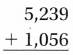Explanation:
The estimated value of the sum is 5000 + 1000 = 6000

Question 2.
Estimate: ____________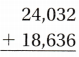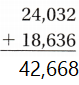Explanation:
The estimated value of the sum is 24000 + 18000 = 42000

Question 3.
Estimate: ____________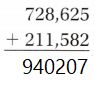Explanation:
The estimated value of the sum is 720,000 + 210,000 = 930,000

Estimate: ____________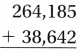Explanation:
The estimated value of the sum is 260,000 + 38,000 = 298,000

Estimate. Then find the difference.

Question 5.
Estimate: ____________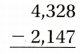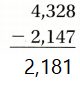Explanation:
The estimated value of the difference is 4000 – 2000 = 2000

Question 6.
Estimate: ____________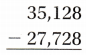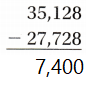Explanation:
The estimated value of the difference is 35000 – 27000 = 7000

Question 7.
Estimate: ____________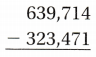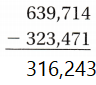Explanation:
The estimated value of the difference is 630,000 – 320,000 = 310,000

Question 8.
Estimate: ____________Explanation:
The estimated value of the difference is 170,000 – 28,000 = 142,000

Find the sum.

Question 9.Explanation:
Found The sum of algebraic equation

Question 10.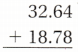Explanation:
Found The sum of algebraic equation

Question 11.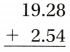Explanation:
Found The sum of algebraic equation

Question 12.
14.36 + 7.87 ___________
14.36 + 7.87 = 22.23
Explanation:
Found The sum of algebraic equation

Question 13.
0.25 + 0.77 ___________
0.25 + 0.77 = 1.02
Explanation:
Found The sum of algebraic equation

Question 14.
6.75 + 8.49 _________
6.75 + 8.49 = 15.24
Explanation:
Found The sum of algebraic equation

Find the difference.

Question 15.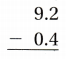Explanation:
Found The difference between algebraic equation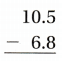Explanation:
Found The difference of algebraic equation

Question 17.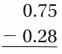Explanation:
Found The difference of algebraic equation

Question 18.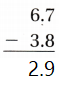Explanation:
Found The difference of algebraic equation

Question 19.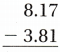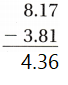Explanation:
Found The difference of algebraic equation

Question 20.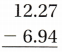Explanation:
Found The difference of algebraic equation

Question 21.
During October, Chad’s website had 12,014 visitors and Pamela’s website had 11,987 visitors. Kim’s website had 6,347 more visitors than Pamela’s website. How many visitors did all three websites have during October?
(A) 30,348
(B) 5,667
(C) 42,335
(D) 18,334
Explanation:
12,014 + 11,987 = 24001
11,987 + 6,347 = 18334
12,014 + 11,987 + 18334 = 42,335 visitors that all three websites have during October

Question 22.
The town of Spring Lake has a population of 30,155 during the summer, a population of 13,876 during the winter, and a population of 22,654 during the fall. How many more people spend time in Spring Lake during the summer and winter combined than during the fall?
(A) 8,778
(B) 44,031
(C) 7,501
(D) 21,377
Explanation:
30,155 + 13,876  = 44031
44031 – 22,654 = 21377 more people spend time in Spring Lake during the summer and winter combined than during the fall

The Smiths are on a summer road trip. They travel 10.9 hours on the first day, 8.6 hours on the second day, and 12.4 hours on the final day. How many hours does the Smith family travel during the three-day trip?
(A) 31.9 hours
(B) 3.8 hours
(C) 23.3 hours
(D) 19.5 hours
Explanation:
10.9 + 8.6 + 12.4 = 31.9 hours that the Smith family traveled during the three-day trip

Question 24.
During one week, Morgan jogged 51.2 kilometers, Karen jogged 53.52 kilometers, and Kelly jogged 6 kilometers less than Morgan. How many more kilometers did Karen jog that week than Kelly?
(A) 104.72 kilometers
(B) 8.32 kilometers
(C) 45.2 kilometers
(D) 57.2 kilometers